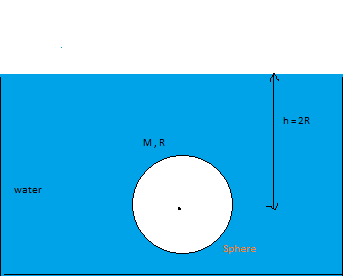# Sphere in Swimming PoolFind The net upward Force exerted by the liquid of an Swimming Pool on the uniform sphere on bottom half sphere. Neglect atmospheric pressure . If Radius of sphere is 'R' and it is at the depth 'h' from the upper upper surface of swimming pool. And density of liquid is $\rho$ It can be Express as : ${ F }_{ upward }\quad =\quad \cfrac { a }{ b } \pi \rho g{ R }^{ c }$

Find a + b + c

###### This is part of My Set Deepanshu's Mechanics Blast
×

Problem Loading...

Note Loading...

Set Loading...